Laplace Transform - 2

# Laplace Transform - 2 | Physics for IIT JAM, UGC - NET, CSIR NET

 1 Crore+ students have signed up on EduRev. Have you?

Fundamental relationships

Since an ordinary Laplace transform can be written as a special case of a two-sided transform, and since the two-sided transform can be written as the sum of two one-sided transforms, the theory of the Laplace-, Fourier-, Mellin-, and Z-transforms are at bottom the same subject. However, a different point of view and different characteristic problems are associated with each of these four major integral transforms.

Table of selected Laplace transforms

The following table provides Laplace transforms for many common functions of a single variable. For definitions and explanations, see the Explanatory Notes at the end of the table.
Because the Laplace transform is a linear operator:

• The Laplace transform of a sum is the sum of Laplace transforms of each term.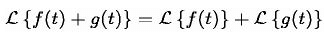• The Laplace transform of a multiple of a function is that multiple times the Laplace transformation of that function.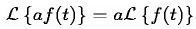Using this linearity, and various trigonometric, hyperbolic, and complex number (etc.) properties and/or identities, some Laplace transforms can be obtained from others quicker than by using the definition directly.
The unilateral Laplace transform takes as input a function whose time domain is the non-negative reals, which is why all of the time domain functions in the table below are multiples of the Heaviside step function, u(t). The entries of the table that involve a time delay τ are required to be causal (meaning that τ > 0). A causal system is a system where the impulse response h(t) is zero for all time t prior to t = 0. In general, the region of convergence for causal systems is not the same as that of anticausal systems.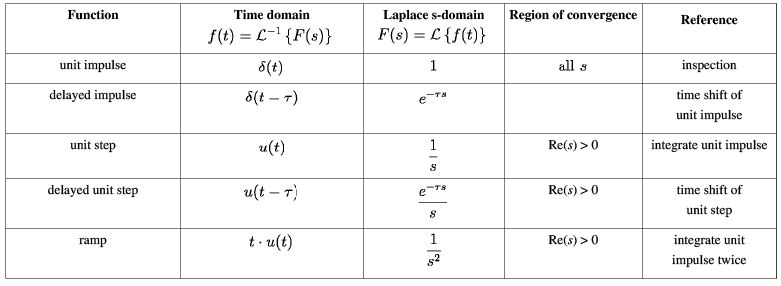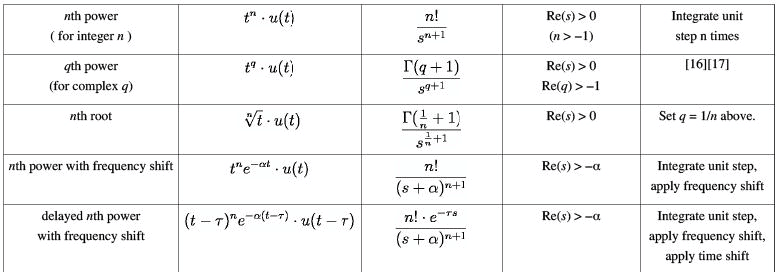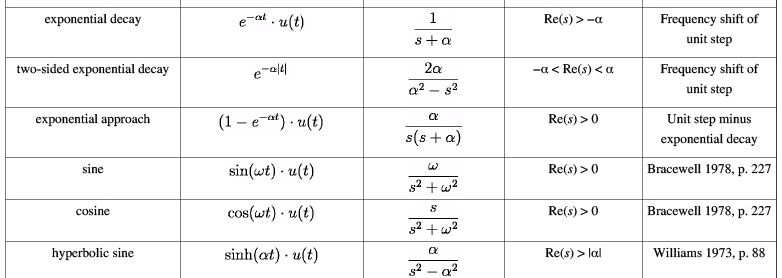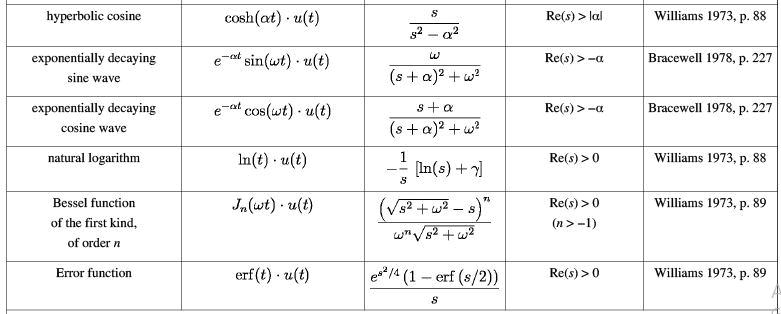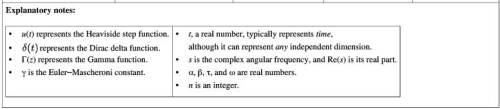s-Domain equivalent circuits and impedances

The Laplace transform is often used in circuit analysis, and simple conversions to the s-Domain of circuit elements can be made. Circuit elements can be transformed into impedances, very similar to phasor impedances.
Here is a summary of equivalents: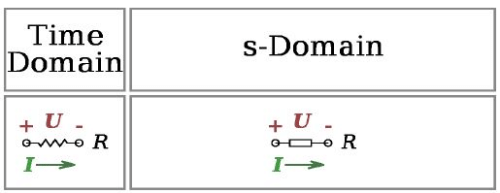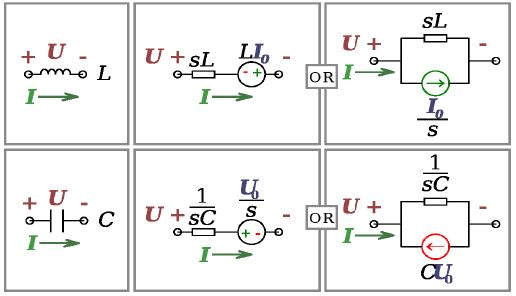Note that the resistor is exactly the same in the time domain and the s-Domain. The sources are put in if there are initial conditions on the circuit elements. For example, if a capacitor has an initial voltage across it, or if the inductor has an initial current through it, the sources inserted in the s-Domain account for that.
The equivalents for current and voltage sources are simply derived from the transformations in the table above.

Examples: How to apply the properties and theorems

The Laplace transform is used frequently in engineering and physics; the output of a linear time invariant system can be calculated by convolving its unit impulse response with the input signal. Performing this calculation in Laplace space turns the convolution into a multiplication; the latter being easier to solve because of its algebraic form. For more information, see control theory.

The Laplace transform can also be used to solve differential equations and is used extensively in electrical engineering. The Laplace transform reduces a linear differential equation to an algebraic equation, which can then be solved by the formal rules of algebra. The original differential equation can then be solved by applying the inverse Laplace transform. The English electrical engineer Oliver Heaviside first proposed a similar scheme, although without using the Laplace transform; and the resulting operational calculus is credited as the Heaviside calculus.

Example 1: Solving a differential equation

In nuclear physics, the following fundamental relationship governs radioactive decay: the number of radioactive atoms N in a sample of a radioactive isotope decays at a rate proportional to N. This leads to the first order linear differential equation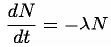where λ is the decay constant. The Laplace transform can be used to solve this equation.
Rearranging the equation to one side, we have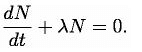Next, we take the Laplace transform of both sides of the equation: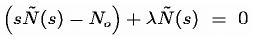where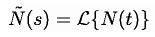and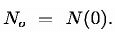Solving, we find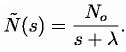Finally, we take the inverse Laplace transform to find the general solution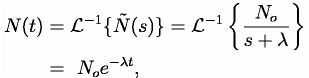which is indeed the correct form for radioactive decay.

Example 2: Deriving the complex impedance for a capacitor

In the theory of electrical circuits, the current flow in a capacitor is proportional to the capacitance and rate of change in the electrical potential (in SI units). Symbolically, this is expressed by the differential equation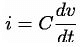where C is the capacitance (in farads) of the capacitor, i = i(t) is the electric current (in amperes) through the capacitor as a function of time, and v = v(t) is the voltage (in volts) across the terminals of the capacitor, also as a function of time.
Taking the Laplace transform of this equation, we obtain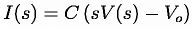where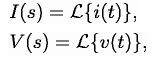and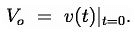Solving for V(s) we have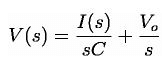The definition of the complex impedance Z (in ohms) is the ratio of the complex voltage V divided by the complex current I while holding the initial state V o at zero: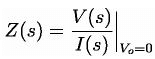Using this definition and the previous equation, we find: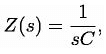which is the correct expression for the complex impedance of a capacitor.

Example 3: Method of partial fraction expansion

Consider a linear time-invariant system with transfer function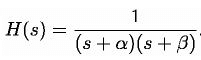The impulse response is simply the inverse Laplace transform of this transfer function: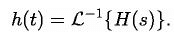To evaluate this inverse transform, we begin by expanding H(s) using the method of partial fraction expansion: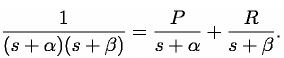The unknown constants P and R are the residues located at the corresponding poles of the transfer function. Each residue represents the relative contribution of that singularity to the transfer function's overall shape. By the residue theorem, the inverse Laplace transform depends only upon the poles and their residues. To find the residue P, we multiply both sides of the equation by s + α to get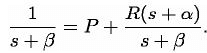Then by letting s = −α, the contribution from R vanishes and all that is left is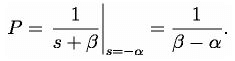Similarly, the residue R is given by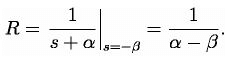Note that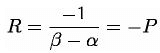and so the substitution of R and P into the expanded expression for H(s) gives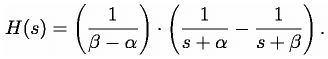Finally, using the linearity property and the known transform for exponential decay (see Item #3 in the Table of Laplace Transforms, above), we can take the inverse Laplace transform of H(s) to obtain: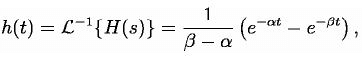which is the impulse response of the system.

Example 3.2: Convolution

The same result can be achieved using the convolution property as if the system is a series of filters with transfer functions of 1/(s+a) and 1/(s+b). That is, the inverse of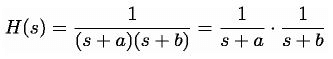is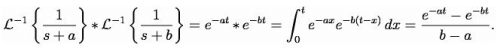Example 4: Mixing sines, cosines, and exponentials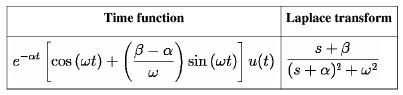Starting with the Laplace transform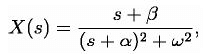we find the inverse transform by first adding and subtracting the same constant α to the numerator: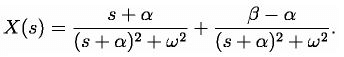By the shift-in-frequency property, we have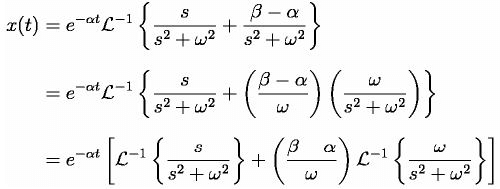Finally, using the Laplace transforms for sine and cosine (see the table, above), we have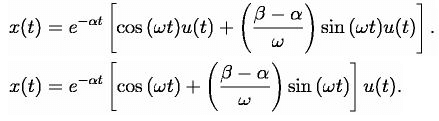Example 5: Phase delay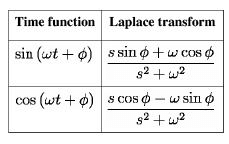Starting with the Laplace transform,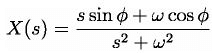we find the inverse by first rearranging terms in the fraction: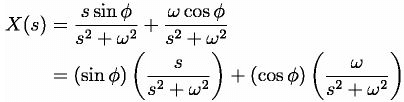We are now able to take the inverse Laplace transform of our terms: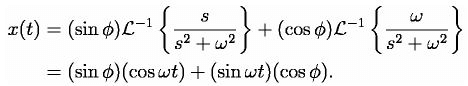This is just the sine of the sum of the arguments, yielding: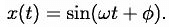We can apply similar logic to find that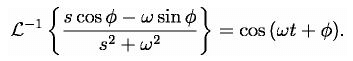Example 6: Determining structure of astronomical object from spectrum

The wide and general applicability of the Laplace transform and its inverse is illustrated by an application in astronomy which provides some information on the spatial distribution of matter of an astronomical source of radiofrequency thermal radiation too distant to resolve as more than a point, given its flux density spectrum, rather than relating the time domain with the spectrum (frequency domain).
Assuming certain properties of the object, e.g. spherical shape and constant temperature, calculations based on carrying out an inverse Laplace transformation on the spectrum of the object can produce the only possible model of the distribution of matter in it (density as a function of distance from the center) consistent with the spectrum. When independent information on the structure of an object is available, the inverse Laplace transform method has been found to be in good agreement.

The document Laplace Transform - 2 | Physics for IIT JAM, UGC - NET, CSIR NET is a part of the Physics Course Physics for IIT JAM, UGC - NET, CSIR NET.
All you need of Physics at this link: Physics

159 docs

## Physics for IIT JAM, UGC - NET, CSIR NET

159 docs

Track your progress, build streaks, highlight & save important lessons and more!(Scan QR code)

,

,

,

,

,

,

,

,

,

,

,

,

,

,

,

,

,

,

,

,

,

,

,

,

,

,

,

;